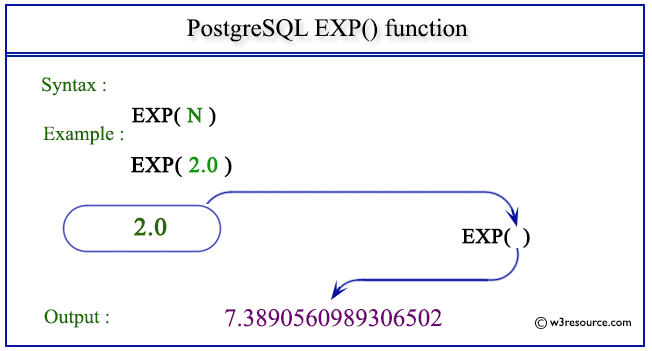# PostgreSQL EXP() function

## EXP() function

The PostgreSQL exp() function is used to return the exponentiation of a number as specified in the argument.

Syntax:

```exp()
```

PostgreSQL Version: 9.3

Pictorial presentation of PostgreSQL EXP() functionExample: PostgreSQL EXP() function:

Code:

``````SELECT EXP(2.0) AS "Exponential";
```
```

Sample Output:

```    Exponential
--------------------
7.3890560989306502
(1 row)
```

Previous: DIV function
Next: FLOOR function

﻿